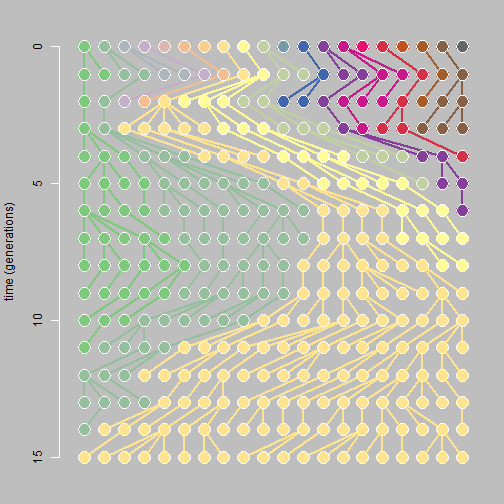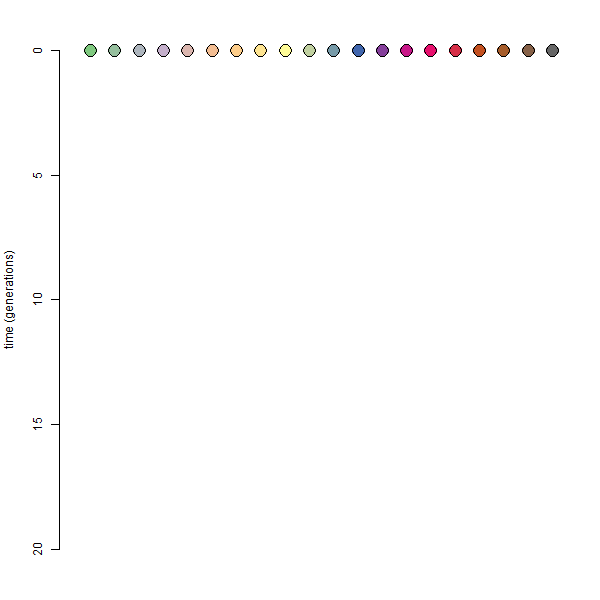## Tuesday, March 6, 2018

### Coalescent genealogy plot in R

Nowadays every evolutionary genetics seems to feature the same plot illustrating genealogical coalescence. You know the one - it usually consists of different colored circles or squares connected by lines meant to represent parent-daughter relationships? I thought it would be handy to reproduce this in R. Here's my function which will shortly join my PopGen package on GitHub:

``````coalescent.plot<-function(n=10,ngen=20,colors=NULL,...){
if(hasArg(sleep)) sleep<-list(...)\$sleep
else sleep<-0.2
if(is.null(colors)) colors<-rainbow(n=n)
popn<-matrix(NA,ngen+1,n)
parent<-matrix(NA,ngen,n)
popn[1,]<-1:n
for(i in 1:ngen){
parent[i,]<-sort(sample(1:n,replace=TRUE))
popn[i+1,]<-popn[i,parent[i,]]
}
plot.new()
par(mar=c(2.1,4.1,2.1,1.1))
plot.window(xlim=c(0.5,n+0.5),ylim=c(ngen,0))
axis(2)
title(ylab="time (generations)")
cx.pt<-2*25/max(n,ngen)
points(1:n,rep(0,n),bg=colors,pch=21,cex=cx.pt)
for(i in 1:ngen){
dev.hold()
for(j in 1:n){
lines(c(parent[i,j],j),c(i-1,i),lwd=2,
col=colors[popn[i+1,j]])
}
points(1:n,rep(i-1,n),bg=colors[popn[i,]],pch=21,
cex=cx.pt)
points(1:n,rep(i,n),bg=colors[popn[i+1,]],pch=21,
cex=cx.pt)
dev.flush()
Sys.sleep(sleep)
}
}
``````

OK, let's try it!

``````library(RColorBrewer)
n<-20
cols<-colorRampPalette(brewer.pal("Accent",n=8))(n)
par(bg="grey",fg="white")
coalescent.plot(n=n,colors=cols,ngen=15)
``````The color scheme is arbitrary & the grey background / white foreground is just for effect - but I like it!

Note that the plot shown is static, but in R it is animated. Here's what I mean:

``````coalescent.plot(n=n,colors=cols,ngen=20)
``````(Note that I also made the above animation using ImageMagick, but I also had to modify the code of `coalescent.plot` to include a call to `dev.print` for every generation of the simulation.)

That's it.

1.1.